# Peripateticism

Yuens' blog

View the Project on GitHub# 基于头部姿态角变换的活体检测

• 配合式：一般用于证券/电话卡等账户开户，用户根据指定动作（眨眼、左右转头、左右摇头、点头）判断活体。如睁眼闭眼（一种做法是对检测得到的人脸的眼睛关键点，抠出眼睛部分，对睁眼闭眼进行分类）；
• 非配合式：用于安防、门禁等场景。就说基于双目摄像头（可见光、红外）的实现原理：可见光和红外摄像头分别采集到三通道彩图和单通道图，将三通道图用于人脸检测，将检测得到的人脸坐标放置在单通道图像上，观察是否有人脸，若有则为活体转而进入后续的任务，如识别，否则就不是活体，可能是一张照片。

## 姿态角/欧拉角动图注解

pitch()：俯仰，将物体绕X轴旋转（localRotationX）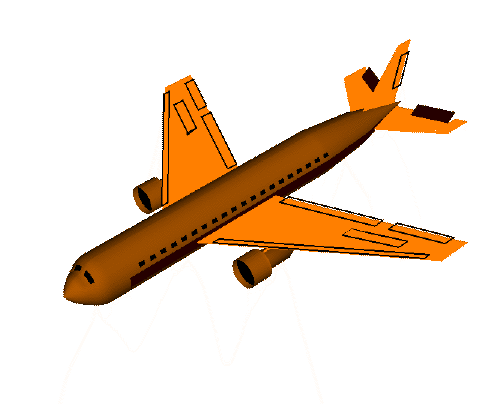yaw()：航向，将物体绕Y轴旋转（localRotationY）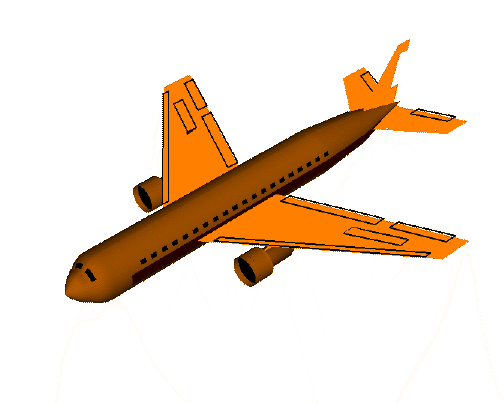roll()：横滚，将物体绕Z轴旋转（localRotationZ）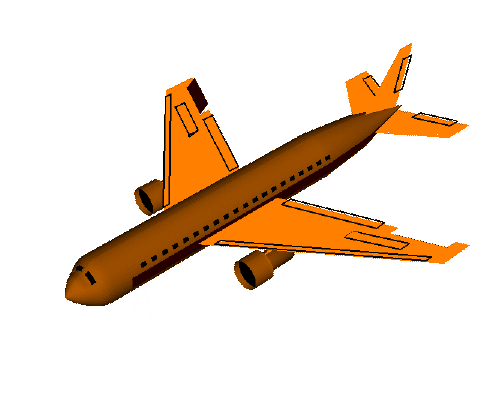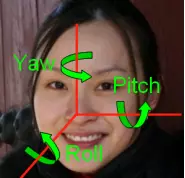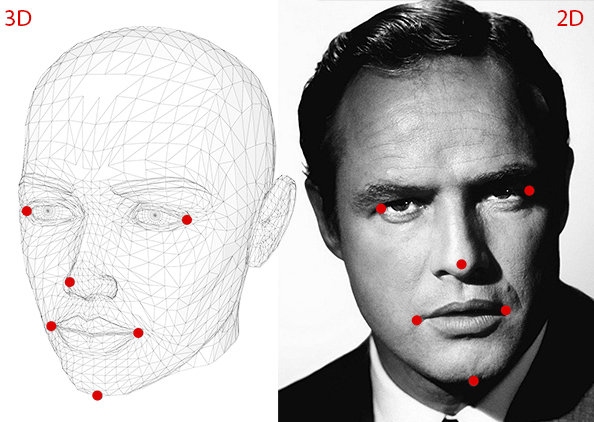1. 物体（人脸）在相机3D世界中投影到二维平面的坐标。即图像上人脸的关键点，比方我们这里是五个关键点或者六个，那么就要给出这几个点在二维平面上的坐标。因为我们已有模型计算得到的人脸关键点信息（这个也可以用Dlib/OpenCV得到，Dlib提供计算人脸68个关键点的模型与接口），所以这个是已知的。
2. 物体与2D平面相对应的3D世界坐标系位置。可能你会认为需要照片中人物的3D模型才能获得3D位置。理想情况下是的，但在实践中我们一是没有，二是通用3D模型就足够了。获得完整头部的3D模型，只需要知道在某个任意参考系中的几个点的3D位置。下面我们以鼻子为3D世界坐标系的原点位置（0，0，0）。得到以下人脸3D点（以下几个点是任意参考系/坐标系中，你还可以根据自己情况修改校准，这称为世界坐标，也即OpenCV docs中的模型坐标）：
• 鼻尖：（0.0,0.0,0.0）
• 下巴：（0.0，-330.0，-65.0）
• 左眼左角：（ - 225.0f，170.0f，-135.0）
• 右眼右角：（225.0,170.0，-135.0）
• 口的左角：（ - 150.0，-150.0，-125.0）
• 嘴角：（150.0，-150.0，-125.0）
3. 物体在3D世界中对应相机中3D坐标系的位置，知道了3D世界中的坐标点通过相机参数可以算出，换句话说，这一步是要知道相机参数（相机焦距、图像中的光学中心、径向失真参数）。因为这不是软件层面的事情，所以我们这里假设相机被校准，使用校准相机的参数。我们通过图像的中心来近似光学中心，以像素的宽度近似焦距，并假设不存在径向畸变。

## 2. 如何在数学上表示物体相对相机的运动？

• 平移（Translation）：将相机从当前3D位置（X，Y，Z）移动到新的3D位置（X'，Y'，Z'）称为平移，平移具有3个自由度，即可以沿X，Y或Z方向移动。平移由向量t表示（X' - X，Y' - Y，Z' - Z）
• 旋转（Rotation）：可以旋转摄像头的X、Y和Z轴，即旋转也具有三个自由度。有许多表示旋转的方法，常见的如欧拉角（包含滚动角，俯仰角和偏航角，对应roll、pitch、和yaw），即一个3x3维度的旋转矩阵或者3x1维度的旋转向量，或者用一个旋转方向（即轴）和角度来定义。

## 3. 姿势估计算法如何工作？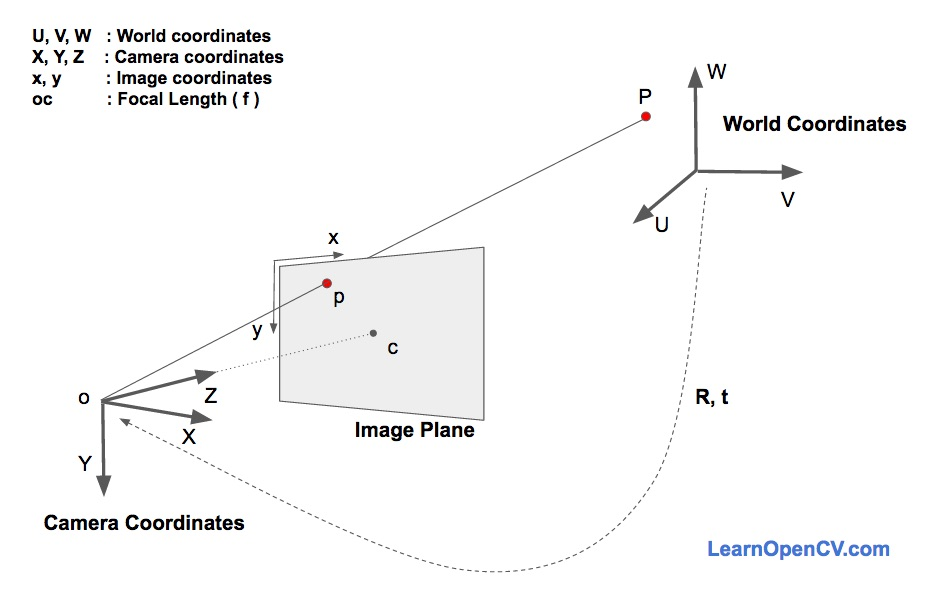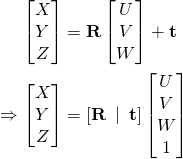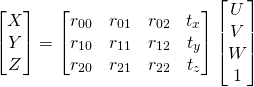## 3.1 方法1：直接线性变换（DLT）求解参数

PS：下面公式描述了，将相机坐标（X，Y，Z）转换即投影为平面坐标（x，y，1）的过程，已知平面坐标和相机参数，通过下面公式算出相机坐标（X，Y，Z）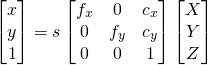s是一个我们未知的比例因子，表示深度信息。举个例子，若将3D世界坐标中某个点P与3D相机坐标中对应的点P连接起来，即光线，那么这个光线会与图像平面相交得到二维平面上的点P。这个s就是这个过程中变换的深度信息，但我们这里因为未知，统统将所有点s考虑为一样的。

PS：以下公式，将3D世界坐标（U，V，W）转换为3D相机坐标，已知3D世界坐标和上面公式算出的3D相机坐标，因而可以算出3x3的旋转矩阵和3x1的平移向量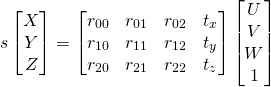## 3.2 方法2：Levenberg-Marquardt

1. 旋转R有3个自由度需要的只是3个数字，但DLT方法使用的矩阵有9个数字，且DLT方法中没有任何方法使3x3矩阵的意义是旋转矩阵（这里我有点懵，可能是说，3x3维度的这个矩阵其实际意义很难说带有旋转的意思在里面，因为没有最小化目标函数，这个算出的3x3的矩阵与旋转的参数意义不明确）；
2. 直接线性变换（DLT）方法不会最小化正确的目标函数，我们希望最小化下面描述中的投影误差。OpenCV的APIsolvePnPPnP，Perspective-n-Point，即从3D点找到其对应的2D点），可以使用基于LM方法（Levenberg-Marquardt）进行姿态估计，只需要定义flag参数为CV_ITERATIVE即可（当然还有其他方法，见后文API说明），无论哪种方法的使用，都需要定义这三个参数：

1. 3D坐标中的点objectPoints
2. 与3D点对应的2D坐标点imagePoints。如人脸关键点位置信息（五点如左右眼睛、嘴唇左右、鼻子）
3. 摄像机的畸变参数distCoeffs（一般是固定的，其中定义了焦距，光学中心等）。确保或者以该参数校准相机，不会有误差、错误的结果等。

## solvePnP

Finds an object pose from 3D-2D point correspondences.

• C++: bool solvePnP(InputArray objectPoints, InputArray imagePoints, InputArray cameraMatrix, InputArray distCoeffs, OutputArray rvec, OutputArray tvec, bool useExtrinsicGuess=false, int flags=ITERATIVE )
• Python: cv2.solvePnP(objectPoints, imagePoints, cameraMatrix, distCoeffs[, rvec[, tvec[, useExtrinsicGuess[, flags]]]]) → retval, rvec, tvec

### Parameters:

• objectPoints – Array of object points in the object coordinate space, 3xN/Nx3 1-channel or 1xN/Nx1 3-channel, where N is the number of points. vector can be also passed here.
• imagePoints – Array of corresponding image points, 2xN/Nx2 1-channel or 1xN/Nx1 2-channel, where N is the number of points.vector can be also passed here.
• cameraMatrix – Input camera matrix  .
• distCoeffs – Input vector of distortion coefficients  of 4, 5, or 8 elements. If the vector is NULL/empty, the zero distortion coefficients are assumed.
• rvec – Output rotation vector (see Rodrigues() ) that, together with tvec , brings points from the model coordinate system to the camera coordinate system.
• tvec – Output translation vector.useExtrinsicGuess – If true (1), the function uses the provided rvec and tvec values as initial approximations of the rotation and translation vectors, respectively, and further optimizes them.
• flags –Method for solving a PnP problem:
1. CV_ITERATIVE Iterative method is based on Levenberg-Marquardt optimization. In this case the function finds such a pose that minimizes reprojection error, that is the sum of squared distances between the observed projections imagePoints and the projected (using projectPoints() ) objectPoints .
2. CV_P3P Method is based on the paper of X.S. Gao, X.-R. Hou, J. Tang, H.-F. Chang “Complete Solution Classification for the Perspective-Three-Point Problem”. In this case the function requires exactly four object and image points.
3. CV_EPNP Method has been introduced by F.Moreno-Noguer, V.Lepetit and P.Fua in the paper “EPnP: Efficient Perspective-n-Point Camera Pose Estimation”. The function estimates the object pose given a set of object points, their corresponding image projections, as well as the camera matrix and the distortion coefficients. Note: An example of how to use solvePNP for planar augmented reality can be found at opencv_source_code/samples/python2/plane_ar.py

## 4. Levenberg-Marquardt优化算法

【】 【】【】【】【】【】【】【】【】【】【】【】【】【】

## 5. 旋转参数转换为欧拉角

1. 旋转向量rotation_vector ——> 四元数（quaterniond，即w,x,y,z）；
2. 四元数（quaterniond，即w,x,y,z）——>对应的欧拉角（pitch、yaw、roll）。

### 5.1 旋转向量（角轴）转为四元数

• q = [cos(\theta / 2), n_x sin(\theta / 2), n_y sin(\theta / 2), n_z sin(\theta / 2)] ^ T
• q = [w, x, y, z]^T
• 其中有|q|^2 = w^2 + x^2 + y^2 + z^2 = 1

# calculate quaterniond from rotation vector
theta = cv2.norm(rotation_vector)
w = np.cos(theta / 2)
x = np.sin(theta / 2) * rotation_vector / theta
y = np.sin(theta / 2) * rotation_vector / theta
z = np.sin(theta / 2) * rotation_vector / theta


### 5.2 四元数转为欧拉角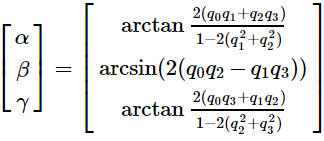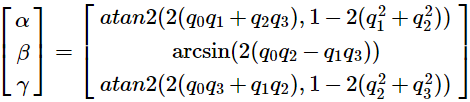# quaterniond to eulerAngle
ysqr = y * y
xsqr = x * x
zsqr = z * z

# pitch (x-axis rotation)
t0 = 2.0 * (w * x + y * z)
t1 = 1.0 - 2.0 * (xsqr + ysqr)
pitch = math.atan2(t0, t1)
pitch = pitch * 180 / math.pi

# yaw (y-axis rotation)
t2 = 2.0 * (w * y - z * x)
t2 = 1.0 if t2 > 1.0 else t2
t2 = -1.0 if t2 < -1.0 else t2
yaw = math.asin(t2)
yaw = yaw * 180 / math.pi

# roll (z-axis rotation)
t3 = 2.0 * (w * z + x * y)
t4 = 1.0 - 2.0 * (ysqr + zsqr)
roll = math.atan2(t3, t4)
roll = roll * 180 / math.pi
if roll > 90:
roll = (roll - 180) % 180
if roll < -90:
roll = (roll + 180) % 180


• 角度转弧度：π/180×角度
• 弧度变角度：180/π×弧度

## 6. 欧拉角与头部姿态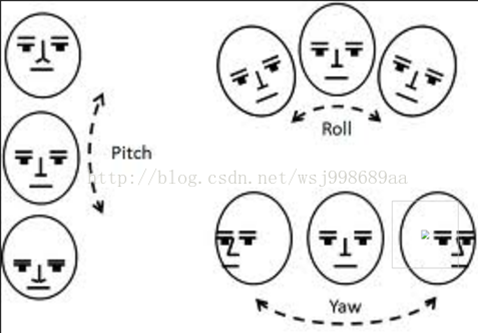1. 从左到右水平转头的一系列图片；
2. 从左到右摇头的一系列图片；
3. 从上到下（抬头到点头）的一系列图片。

1. 从左到右水平转头的一系列图片的欧拉角，即yaw，pitch，roll的角度变化范围；
2. 从左到右摇头的一系列图片的欧拉角，即yaw，pitch，roll的角度变化范围；
3. 从上到下（抬头到点头）的一系列图片的欧拉角，即yaw，pitch，roll的角度变化范围。

def get_pose(self, angle_dict):
pose_dict = {'down':0,'front':0,\
'turn_left':0, 'turn_right':0,\
'shake_left':0, 'shake_right':0}
# pitch
if -180<angle_dict['pitch'] and angle_dict['pitch']<-100:
pass #sose_dict['down'] = 1
elif 100<angle_dict['pitch'] and angle_dict['pitch']<180:
pose_dict['up'] = 1
# yaw
if -70<angle_dict['yaw'] and angle_dict['yaw']<-15:
pose_dict['turn_left'] = 1
elif 15<angle_dict['yaw'] and angle_dict['yaw']<70:
pose_dict['turn_right'] = 1
# roll
if 10<angle_dict['roll']<70:
pose_dict['shake_left'] = 1
elif -70<angle_dict['roll']<-10:
pose_dict["shake_right"] = 1
# front
if self.is_front(angle_dict):
pose_dict["front"] = 1
return pose_dict

def is_front(self, angle_dict):
if abs(angle_dict['yaw'])<10 and\
abs(angle_dict['roll'])<10 and\
abs(angle_dict['pitch'])>160:
return True
return False